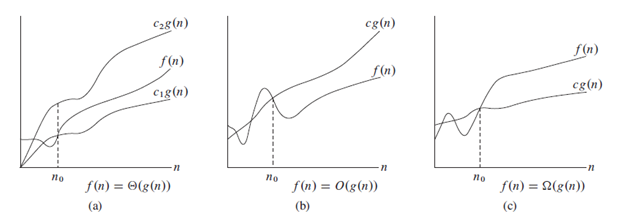# Introduction to asymptotic notation with detailed description on theta(Θ), big-oh(O), omega(Ω), little-oh(o) and little-omega(ω) notations

 Category: Data Structure And Algorithms Tags:

## Introduction

Anyone who starts studying algorithms the first thing comes in picture is asymptotic notations, these notations are used to define complexity of an algorithm. The running time of any algorithm can be represented in terms of asymptotic notations which is called time complexity of a function.

The complexity depends on the input size and we represent input size as n (i.e. size of an input array) and give complexity in terms of n like O(n), O(log n) etc.

## Θ – Notation

As in previous article of data structures and algorithm section we analyzed insertion sort which complexity was Θ(n2). Let’s suppose a complexity function g(n) which complexity given by Θ(g(n)) (that is nothing but set of functions), the definition is:

Θ(g(n)) = { f(n) : there exist positive constants c1,c2 and n0 such that

0 ≤ c1g(n)≤ f(n) ≤ c2g(n) for all n ≥ n0 }In above pictures, graph(a) presents Θ(theta) notation which says there will be function f(n) which will always fall between/on c1g(n) and c2g(n) when n≥n0. Here c1, c2, n0 are constants, and we can see in graph at  x = n0 where y = f(n) always falls between y=c1g(n) and y=c2g(n).

Above we said Θ(g(n)) is nothing but set of functions so that f(n) Θ(g(n)) means f(n) is member of Θ(g(n))but we usually write it like f(n) = Θ(g(n)) here g(n) is the function which is called asymptotically tight bound for f(n). If we say c1n ≤ (n+5) ≤ c2n so here that will be always true for some values of c1 and c2.

In terms of algorithmic analysis, Θ can be defined combination of worst and best case complexity because it defines the lower and upper boundaries, so lower boundary can be called as best case and upper can be as worst case.

## O – Notation

Big-oh notation (O notation) is widely used notation because it defines worst case or we can say it presents asymptotically upper bound function. The definition is:

O(g(n)) = { f(n) : there exist positive constants c,n0 such that

0 ≤ f(n) ≤ cg(n) for all n ≥ n0 }

O notation only gives upper bound so Θ is more powerful notation than O. Same as Θ we can say f(n) O(g(n)) and so Θ(g(n))O(g(n)). For any quadratic function ax2 + bx + c we can say complexity will be O(n2) because x2 is the biggest term if you take it out as x2(a+ b/x + c/x2) then for large values of x, the part in brackets will become very less in front of x2. As shown in figure(b) above we can see after n0 the function cg(n) is always above then f(n).

## Ω - Notation

As O notation provides upper bound Ω(big-omega or omega) notation will bound a function with lower boundaries. It provides asymptotically lower bound, the definition is:

Ω(g(n)) = { f(n) : there exist positive constants c, n0 such that

0 ≤ cg(n) ≤ f(n) for all n ≥ n0 }

Ω -notation is used for best case, because it shows minimum running time for any algorithm by its lower boundary. This says the running for any algorithm with any input size n, the minimum running time will be cg(n) as shown in figure(c) above.

## o – Notation

Little oh notation (o notation) represents upper bound but may or may not asymptotically tight. For example the running time 5n we may write complexity o(n2). But for big oh notation complexity of 5n will be O(n) only. Little oh notation will denote upper bound which is not asymptotically tight, definition is:

o(g(n)) = { f(n) : there exist positive constants c> 0,n0> 0 such that

0 ≤ f(n) < cg(n) for all n ≥ n0 }

For example for 5n, T(n) = o(n2) but T(n) ≠ o(n).

## ω – Notation

Little omega notation (ω notation) is for big omega as same as little oh for big oh. The little omega shows lower bound which is not asymptotically tight, means 5n may be written as O(1). The definition is:

ω(g(n)) = { f(n) : there exist positive constants c>0, n0>0 such that

0 ≤ cg(n) <f(n) for all n ≥ n0 }

 Like2 PeopleNikhil Joshi Ceo & Founder at Dotnetlovers Atricles: 165 Questions: 16 Given Best Solutions: 16 *

### Reference:

Introduction to Algorithms, CLRSNice Explanation
Like1 Person on 24 January 2016

Login via:x